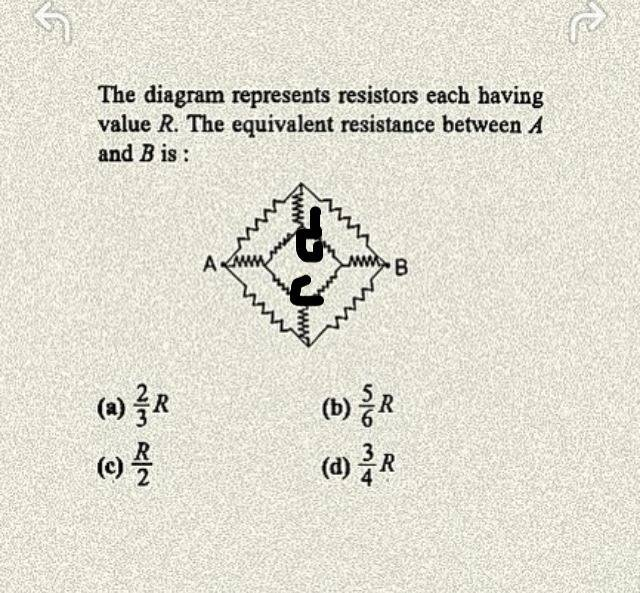# Equivalent resistance of a complicated circuitI know that the current should flow through all the circuit but I have heard that the two vertical R in the circuit don't count I can't understand why?!! I need a obvious clear explanation for this in detail thanks in advance.

Simon Bridge
Homework Helper
I need a obvious clear explanation for this in detail thanks in advance.
You would probably like one - but this is homework so you may not get anything that simple even if it is available. What you need is the tools to figure it out for yourself.

The very simplest reason a resistor may not count is if the potential difference across it is zero.
You can probably see what happens if you redraw the circuit into a box shape as opposed to the current diamond shape.

You could also try putting an arbitrary potential difference across A and B, then using kirkoffs laws.

This sort of exercise is set to test how well you understand electric circuits - with experience, painful experience I'm afraid, you will get so you can look at the circuit and see that this or that situation must hold. Going through the process from scratch is how you get the understanding in the first place.

Last edited:
I don't understand the nature of flow of electrons, if There are two points connected, each point has a current of equal potential, shouldn't each current act independently, why do I calculate the potential difference between two different current

Simon Bridge
Homework Helper
If you attach a battery (say) across A and B, you are creating an electric field throughout the circuit.
The electric field can be described by the potential at different places in the circuit.
The free charges in the material respond to the electric field and produce a current.

Electric current flows from higher potential to lower potential in proportion to potential difference and inversely proportional to the resistance. I=V/R

It follows that if the potential difference across a component is zero - then the current through the component will also be zero.

You do not calculate the potential difference between two different currents - you calculate it between two different places. Those places may or may not have current flowing through them - that is irrelevant.

So when is the potential difference between two points equals zero ??

You can use star-delta transformation technique.
Or as Dr. Simon Bridge pointed out,you can prove that the potential difference is zero.
For proving that the potential difference is zero, take the first half of the network. Clearly they form the wheatstone bridge condition. Thus no current flows through the vertical resistor.

You can use star-delta transformation technique.
Or as Dr. Simon Bridge pointed out,you can prove that the potential difference is zero.
For proving that the potential difference is zero, take the first half of the network. Clearly they form the wheatstone bridge condition. Thus no current flows through the vertical resistor.

Please could you be more specific?

I worked it out. You just need to work on the upper part(first eight resistors) of the circuit.
There are two independent loops(one on left and one on right). Assign a potential difference across each of the eight resistors. You could see that there are three loops. Write the Kirchoff's voltage laws for the loops. You can easily prove that potential difference is zero.

When potential difference is zero, potential is same. When potential is same, no current flows. So the resistor becomes dummy.

CWatters
Homework Helper
Gold Member
Sharan has given you the answer.

In this problem all the resistors have the same value so you can identify pairs of nodes that would be at the same potential by looking at the symmetry of the circuit.

If you have two nodes at exactly the same voltage you can connect them together with a wire without altering the circuit operation. Such wires may make some resistors redundant.

Simon Bridge